×#### Thank you for registering.

One of our academic counsellors will contact you within 1 working day.

Click to Chat

1800-1023-196

+91-120-4616500

CART 0

• 0

MY CART (5)

Use Coupon: CART20 and get 20% off on all online Study Material

ITEM
DETAILS
MRP
DISCOUNT
FINAL PRICE
Total Price: Rs.

There are no items in this cart.
Continue Shopping• Complete JEE Main/Advanced Course and Test Series
• OFFERED PRICE: Rs. 15,900
• View Details

```Chapter 2: Polynomials Exercise – 2.3

Question: 1

Apply division algorithm to find the quotient q(x) and remainder r(x) on dividing f(x) by g(x) in each of the following:

(i) f(x) = x3 – 6x2 + 11x – 6, g(x) = x2 + x + 1

(ii) f(x) = 10x4 + 17x3 – 62x2 + 30x – 105, g(x) = 2x2 + 7x + 1

(iii) f(x) = 4x3 + 8x2 + 8x + 7, g(x) = 2x2 – x + 1

(iv) f(x) = 15x3 – 20x2 + 13x – 12, g(x) = x2 – 2x + 2

Solution:

(i) f(x) = x3 – 6x2 + 11x – 6 and g(x) = x2 + x + 1(ii) f(x) = 10x4 + 17x3 – 62x2 + 30x – 105, g(x) = 2x2 + 7x + 1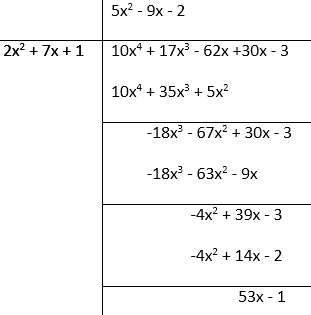(iii) f(x) = 4x3 + 8x2 + 8x + 7, g(x) = 2x2 – x + 1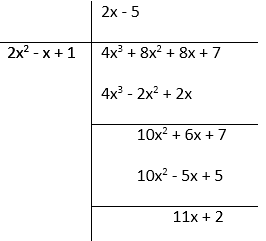(iv) f(x) = 15x3 – 20x2 + 13x – 12, g(x) = x2 – 2x + 2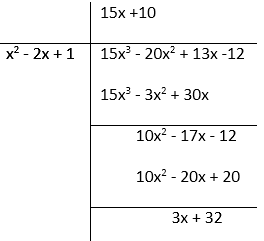Question: 2

Check whether the first polynomial is a factor of the second polynomial by applying the division algorithm:

(i) g(t) = t2 – 3; f(t) = 2t4 + 3t3 – 2t2 – 9t – 12

(ii) g(x) = x2 – 3x + 1; f(x) = x5 – 4x3 + x2 + 3x + 1

(iii) g(x) = 2x2 – x + 3; f(x) = 6x5 − x4 + 4x3 – 5x2 – x – 15

Solution:

(i) g(t) = t2 – 3; f(t) = 2t4 + 3t3 – 2t2 – 9t – 12g(t) = t2 – 3g(t) = t2 – 3 f(t) = 2t4 + 3t3 – 2t2 – 9t Therefore, g(t) is the factor of f(t).

(ii) g(x) = x2 – 3x + 1; f(x) = x5 – 4x3 + x2 + 3x + 1g(x) = x2 – 3x + 1 g(x) = x2 – 3x + 1 f(x) = x5 – 4x3 + x2 + 3x + 1.Therefore, g(x) is not the factor of f(x).

(iii) g(x) = 2x2 – x + 3; f(x) = 6x5 − x4 + 4x3 – 5x2 – x – 15g(x) = 2x2 – x + 3 g(x) = 2x2 – x + 3 f(x) = 6x5 − x4 + 4x3 – 5x2 – x – 15

Question: 3

Obtain all zeroes of the polynomial f(x) = f(x) = 2x4 + x3 – 14x2 – 19x – 6, if two of its zeroes are -2 and -1.

Solution:

f(x) = 2x4 + x3 – 14x2 – 19x – 6

If the two zeroes of the polynomial are -2 and -1, then its factors are (x + 2) and (x + 1)

(x + 2)(x + 1) = x2 + x + 2x + 2 = x2 + 3x + 2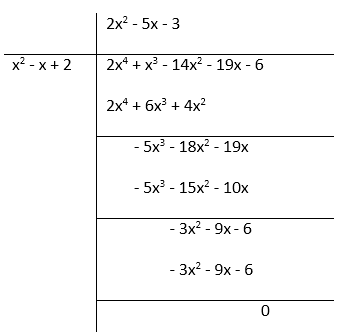f(x) = 2x4 + x3 – 14x2 – 19x – 6 = (2x2 – 5x – 3)(x2 + 3x + 2)

= (2x + 1)(x – 3)(x + 2)(x + 1)

Therefore, zeroes of the polynomial = - 1/2, 3, -2 , -1

Question: 4

Obtain all zeroes of f(x) = x3 + 13x2 + 32x + 20, if one of its zeroes is -2.

Solution:

f(x) = x3 + 13x2 + 32x + 20

Since, the zero of the polynomial is -2 so, it means its factor is (x + 2).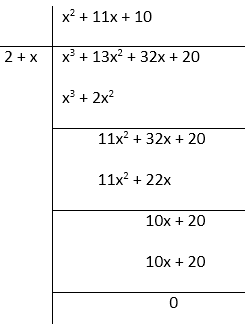So, f(x) = x3 + 13x2 + 32x + 20 =  (x2 + 11x + 10)(x + 2)

= (x2 + 10x + x + 10)(x + 2)

= (x + 10)(x + 1)(x + 2)

Therefore, the zeroes of the polynomial are – 1, – 10, – 2.

Question: 5

Obtain all zeroes of the polynomial f(x) = x4 – 3x3 – x2 + 9x – 6, if the two of its zeroes are – √3 and √3.

Solution:

f(x) = x4 – 3x3 – x2 + 9x – 6 Since, two of the zeroes of polynomial are -√3 and √3 so,(x + √3)(x – √3) = x2– 3x2 – 3So, f(x) = x4 – 3x2 – x2 + 9x – 6 = (x2 – 3)(x2 – 3x + 2)

= (x + √3)(x – √3)(x2– 2x – 2 + 2)

= (x + √3)(x – √3)(x – 1)(x – 2)

Therefore, the zeroes of the polynomial are - √3, √3, 1, 2.

Question: 6

Obtain all zeroes of the polynomial f(x) = 2x4 – 2x3 – 7x2 + x – 1, if the two of its zeroes are – √(3/2) and √(3/2).

Solution:

f(x) = 2x4 – 2x3 – 7x2 + x – 1 Since, - √(3/2)  and √(3/2) are the zeroes of the polynomial, so the factors are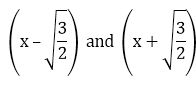So, f(x) = 2x4– 2x3–7x2+ x – 1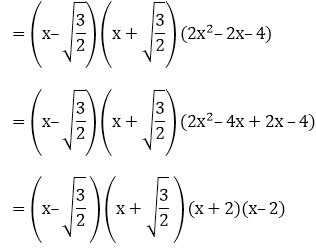Therefore, the zeroes of the polynomial = x = -1, 2, -√(3/2) and √(3/2).

Question: 7

Find all the zeroes of the polynomial x4 + x3 – 34x2 – 4x + 120, if the two of its zeroes are 2 and – 2.

Solution:

x4 + x3 – 34x2 – 4x + 120 Since, the two zeroes of the polynomial given is 2 and – 2 So, factors are (x + 2)(x – 2) =  x2 + 2x – 2x – 4 = x2 – 4x2 – 4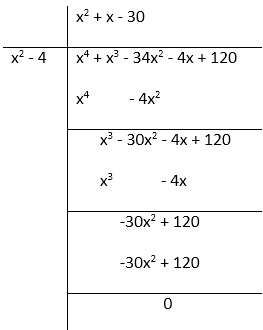So, x4 + x3 – 34x2 – 4x + 120 = (x2 – 4)(x2 + x – 30)

= (x – 2)(x + 2)(x2 + 6x – 5x – 30)

=  (x – 2)(x + 2)(x + 6)(x – 5)

Therefore, the zeroes of the polynomial = x = 2, – 2, – 6, 5

Question: 8

Find all the zeroes of the polynomial 2x4 + 7x3 – 19x2 – 14x + 30, if the two of its zeroes are √2 and - √2.

Solution:

2x4 + 7x3 – 19x2 – 14x + 302 Since, √2 and-√2 are the zeroes of the polynomial given. So, factors are (x + √2) and (x - √2)

= x2+√2x – √2x – 2 = x2 – 2So, 2x4 + 7x3 – 19x2 – 14x + 30 = (x2 – 2)(2x2 + 7x – 15)

= (2x2+ 10x – 3x – 15)(x + √2)(x – √2)

= (2x – 3)(x + 5)(x + √2)(x – √2)

Therefore, the zeroes of the polynomial is √2,- √2,-5, 3/2.

Question: 9

Find all the zeroes of the polynomial f(x) = 2x3 + x2 – 6x – 3, if two of its zeroes are – √3 and √3.

Solution:

f(x) = 2x3 + x2 – 6x – 3 Since, -√3 and √3 are the zeroes of the given polynomial So, factors are (x - √3) and (x + √3)

= (x2– √3x + √3x – 3) = (x2 – 3)So, f(x) = 2x3 + x2 – 6x – 3 = (x2 – 3)(2x + 1)

= (x – √3)(x + √3)(2x + 1)

Therefore, set of zeroes for the given polynomial = √3,– √3, –1/2

Question: 10

Find all the zeroes of the polynomial f(x) = x3 + 3x2 – 2x – 6, if the two of its zeroes are √2 and - √2.

Solution:

f(x) = x3 + 3x2 – 2x – 6 Since, √2 and -√2 are the two zeroes of the given polynomial. So, factors are (x + √2) and (x - √2)

= x2+ √2x – √2x – 2 = x2 – 2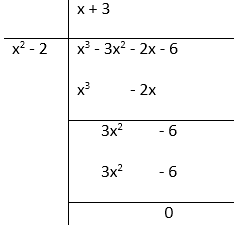By division algorithm, we have:

f(x) = x3 + 3x2 – 2x – 6 = (x2 – 2)(x + 3)

= (x – √2)(x + √2)(x + 3)

Therefore, the zeroes of the given polynomial is - √2, √2 and – 3.

Question: 11

What must be added to the polynomial f(x) = x4 + 2x3 – 2x2 + x − 1 so that the resulting polynomial is exactly divisible by g(x) = x2 + 2x − 3.

Solution:

f(x) = x4 + 2x3 – 2x2 + x − 1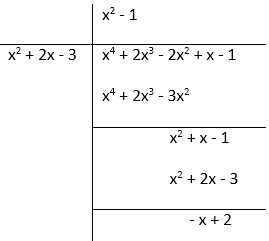We must add (x – 2) in order to get the resulting polynomial exactly divisible by g(x) = x2 + 2x − 3.

Question: 12

What must be subtracted from the polynomial f(x) = x4 + 2x3 – 13x2 –12x + 21 so that the resulting polynomial is exactly divisible by g(x) = x2 – 4x + 3.

Solution:

f(x) = x4 + 2x3 – 13x2 – 12x + 21We must subtract (2x – 3) in order to get the resulting polynomial exactly divisible by g(x) = x2 – 4x + 3.

Question: 13

Given that √2 is a zero of the cubic polynomial f(x) = 6x3+ √2x2– 10x – 4√2, find its other two zeroes.

Solution:

f(x) = 6x3+√2x2 – 10x – 4√2 Since, √2 is a zero of the cubic polynomial So, factor is (x–√2)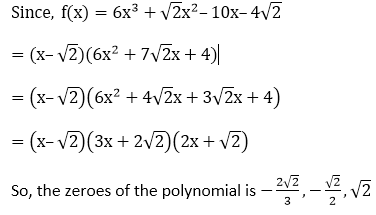Question: 14

Given that x – √5 is a factor of the cubic polynomialfind all the zeroes of the polynomial.

Solution:In the question, it’s given that x – √5 is a factor of the cubic polynomial.So, the zeroes of the polynomial```### Course Features

• 728 Video Lectures
• Revision Notes
• Previous Year Papers
• Mind Map
• Study Planner
• NCERT Solutions
• Discussion Forum
• Test paper with Video Solution